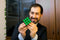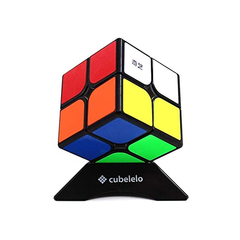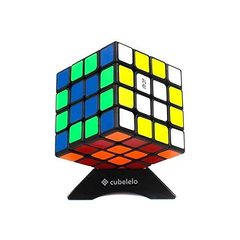After decades, a group of mathematicians, academics, and engineers discovered a number that explains life, the universe, and everything in between. That is the biggest secret of all – the God's Number.  The God's Number is the maximum number of moves required to solve any of the 43 quintillions or to put it in perspective, 43,252,003,274,489,856,000 permutations combinations of the cube. Apart from God Number you can also Rubik's cube under 30 second using F2L method.

Studies and research have proven that God's Number is number 20. This discovery is fairly recent (July 2010). The term God's Number was coined to understand that a mind with the capability of finding the shortest sequence of moves to solve any scramble would have to be so much more powerful than the mind of the common man. Such a mind, mathematicians believe, could be possessed only by a Deity.

This is how you can understand this concept better. It is known that 490 million combinations are required for all the 20 moves to be solved. Even though 490 million seems like a big number, it is only a tiny fraction of the 43 quintillion possible combinations. However, the number of combinations that can be solved in 19 moves is quite closer to 1.5 quintillions.

Therefore, God's Number is much closer to 19 than 20 moves. But even if one scramble sequence was impossible to solve in less than 20 moves, God's Number would still be 20!

## The Super-Flip

In 1995 Michael Reid (mathematician) found a Rubik's Cube configuration called the 'super-flip'. He proved that it requires at least 20 moves to solve. This can be performed using an algorithm.

 R L U2 F U’ D F2 R2 B2 L U2 F’ B’ U R2 D F2 U R2 U 1. Rotate the right face clockwise 2. Rotate the left face clockwise 3. Rotate the front face clockwise twice 4. Rotate the font face clockwise 5. Rotate the up face counterclockwise 6. Rotate the down face clockwise 7. Rotate the front face clockwise twice 8. Rotate the right face clockwise twice 9. Rotate the back face clockwise twice 10. Rotate the left face clockwise 11. Rotate the up face clockwise twice 12. Rotate the front face counterclockwise 13. Rotate the back face counterclockwise 14. Rotate the up face clockwise 15. Rotate the right face clockwise twice 16. Rotate the down face clockwise 17. Rotate the front face clockwise twice 18. Rotate the up face clockwise 19. Rotate the right face clockwise twice 20. Rotate the up face clockwise.

This algorithm will lead to a position wherein all the single corners are solved, and every edge is exactly in its place. This was the first position that was realized could not be solved in less than 20 moves.

If you wish to give it a try, check out this video by RedKB that does a superflip on larger cubes as well.

Many upper bounds, however, were proven over the decades. Morwen Thistlewaite, an early analyst of the mathematical cube, proved that any cube could be solved in 52 moves at the most.Tomas Rockicki, a computer programmer, designed a strategy for finding shorter solutions for Rubik's Cube configurations. His strategy was based on a previous work done by Herbert Kociemba that divided solving a Rubik's Cube into two parts based on a set of 19.5 billion partially-solved configurations with short solutions.

### God's Number for 2x2x2The 2x2x2 Pocket Cube has about 3,674,160 permutations. However, God's Number has been proved to be 11 moves using half-turn metric, or 14 using the quarter-turn metric wherein half turns count as two rotations.

### God's Number for 4x4x4The 4x4x4 Rubik's Revenge has no determined God's Number yet. This is because the centrepiece on the Rubik's Revenge is not fixed. Due to which, parities occur. However, it is estimated that God's Number for the 4x4x4 cube is between 30 and 33.

Recommended ReadHow to Get Faster in Rubik's Cube [Beginners to Pro]

## Conclusion

The strategy for answering the question of God Number took over three decades! It required a set of supercomputers to finally find out answers that suggested that this may not be the ideal approach to solving a cube hobbyist. But this is the kind of problem that mathematicians love to play around with! Since God's Number for the higher cubes (4x4 and beyond) have not yet been known, we should prepare ourselves for more discoveries in the future!